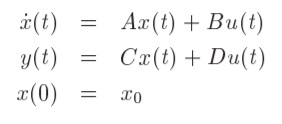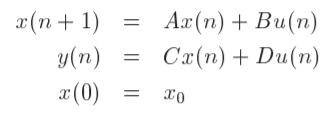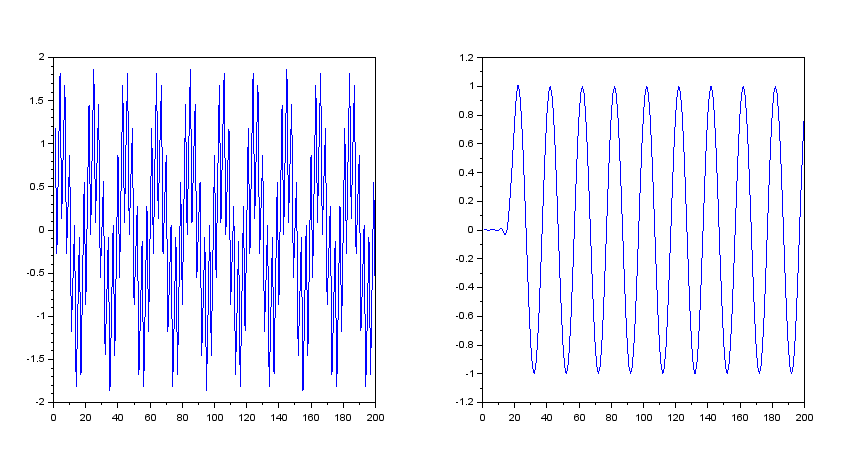# Basic tools for Signal Processing

## Polynomials and System Transfer Functions

Polynomials, matrix polynomials and transfer matrices are also defined and Scilab permits the definition and manipulation of these objects in a natural, symbolic fashion. Polynomials are easily created and manipulated. The poly primitive in Scilab can be used to specify the coefficients of a polynomial or the roots of a polynomial.

```//demonstrate evaluation of discrete filter

//on the unit circle in the z-plane

h=[1:5,4:-1:1];

hz=poly(h,'z','c')

f=(0:.1:1);

hf=freq(hz,1,exp(%pi*%i*f));

hf'```

## State Space Representation

The classical state-space description of a continuous time linear system is :where A, B, C, and D are matrices and x0 is a vector and for a discrete time system takes the formState-space descriptions of systems in Scilab use the syslin function.

## Changing System Representation

Sometimes linear systems are described by their transfer function and sometimes by their state equations. In the event where it is desirable to change the representation of a linear system there exists two Scilab functions which are available for this task. The first function tf2ss converts systems described by a transfer function to a system described by state space representation. The second function ss2tf works in the opposite sense.

`//Illustrate use of ss2tf and tf2ss`
`sl=tf2ss(h)`
`h=ss2tf(sl)`
`h1=iir(3,'lp','butt',[.3 0],[0 0])`
`h1=syslin('d',h1);`
`s1=tf2ss(h1)`

Here the transfer function of a discrete IIR filter is created using the function iir (see Section 4.2). The fourth element of h1 is set using the function syslin and then using tf2ss the state-space representation is obtained in list form.

## Filtering of Signals

Filtering of signals by linear systems (or computing the time response of a system) is done by the function flts which has two formats . The first format calculates the filter output by recursion and the second format calculates the filter output by transform.

`//make signal and filter`
`[h,hm,fr]=wfir('lp',33,[.2 0],'hm',[0 0]);`
`t=1:200;`
`x1=sin(2*%pi*t/20);`
`x2=sin(2*%pi*t/3);`
`x=x1+x2;`
`z=poly(0,'z');`
`hz=syslin('d',poly(h,'z','c')./z**33);`
`yhz=flts(x,hz);`
`subplot(1,2,1)`
`plot(x);`
`subplot(1,2,2)`
`plot(yhz);`